## Introduction

Although, there is lot of support for (ANSI) SQL support in Snowflake there are always the nittygritty things that I always assumed in Microsoft SQL doesn't work in Snowflake. This blogpost is a collection of these differences between Microsoft SQL. This applicable for SQL Server (on premise), Azure SQL Database and Azure Synapse.

## Azure SQL Database to Snowflake conversion issues

There are some differences with the comma between Microsoft SQL and Snowflake. It seems that Microsoft SQL is less strict with commas than Snowflake.

Azure SQL Database  (and other SQL Server versions)

In this example, there is NOT a comma between the NULL and the CONSTRAINT. To my surprise Microsoft SQL code is accepted.

```DROP TABLE IF EXISTS dbo.test;

CREATE TABLE dbo.test(
ID	      VARCHAR (50)  NOT NULL,
Oms       VARCHAR (255)     NULL
CONSTRAINT [PK_ID] PRIMARY KEY NONCLUSTERED (ID ASC)
);

```

Results in :

Snowflake
But when I try this in Snowflake it is not accepted and it results in an error.

```CREATE TABLE test(
ID	      VARCHAR (50)  NOT NULL,
Oms       VARCHAR (255)     NULL
CONSTRAINT PK_ID PRIMARY KEY (ID)
);
```

Results in  :

Azure SQL Database  (and other SQL Server versions)

Another example is the following script. Look at the last line with , . Strange at first sight and I didn't expect that Microsoft SQL accepts kind of code, but surprise surprise, no errors.

```DROP TABLE IF EXISTS dbo.test2;

CREATE TABLE dbo.test2(
ID	      VARCHAR (50)  NOT NULL,
oms       VARCHAR (255)     NULL,
);
```

Results in :

Snowflake
The same code in Snowflake results in an error.

```DROP TABLE IF EXISTS test2;

CREATE TABLE test2(
ID	      VARCHAR (50)  NOT NULL,
oms       VARCHAR (255)     NULL,
);
```

Results in :

## Final thoughts

This is a small blogpost about some experiences with converting Microsoft SQL code (SQL Server, Azure SQL Database and Synapse) to Snowflake.

Hennie

## Introduction

In this blogpost we will explore the date datatypes, date functions and other (date) functionality in Snowflake. This blogpost is broken into several sections that you may like. These are the subjects we would like to discuss :
• It's important to set the timezone properly in your Snowflake account.
• What are the date datatypes in Snowflake? And how can we format the Date data types?
• A bit of arithmetic with date datatypes.
• Several sections about the snowflake Date functions.

## Timezones in Snowflake

The default timezone in Snowflake is 'America/Los_Angeles'. It is preferable to set the time zone to the timezone where you are or where your company exists. You can set the timezone on the account-, session- and object level.

``````SHOW PARAMETERS LIKE '%TIMEZONE%' IN ACCOUNT;      --America/Los_Angeles

ALTER ACCOUNT SET TIMEZONE = 'Europe/Amsterdam';

SHOW PARAMETERS LIKE '%TIMEZONE%' IN ACCOUNT;     --Europe/Amsterdam```
```

Results :

## Snowflake Date Data types

These are the date datatypes that are available in Snowflake:

 Date datatypes Description DATE Snowflake supports a single DATE data type for storing dates (with no time elements). DATETIME DATETIME is an alias for TIMESTAMP_NTZ TIME Snowflake supports a single TIME data type for storing times. TIMESTAMP TIMESTAMP in Snowflake is a user-specified alias associated with one of the TIMESTAMP_* variations (specified by the TIMESTAMP_TYPE_MAPPING session parameter). TIMESTAMP_LTZ TIMESTAMP_LTZ internally stores UTC time with a specified precision. TIMESTAMP_NTZ TIMESTAMP_NTZ internally stores “wallclock” time with a specified precision. TIMESTAMP_TZ TIMESTAMP_TZ internally stores UTC time together with an associated time zone offset.

Here is an example of the date datatypes.

```SELECT
'2021-05-23 12:00:00'::DATE,
'2021-05-23 12:00:00'::DATETIME,
'2021-05-23 12:00:00'::TIMESTAMP,
'12:00:00'::TIME,
'2021-05-23 12:00:00'::TIMESTAMP_LTZ,
'2021-05-23 12:00:00'::TIMESTAMP_NTZ,
'2021-05-23 12:00:00'::TIMESTAMP_TZ;
```

This results in :

You can change the format of the date datatypes with parameters DATE_OUTPUT_FORMAT, DATE_INPUT_FORMAT, TIMESTAMP_INPUT_FORMAT, TIMESTAMP_LTZ_OUTPUT_FORMAT, TIMESTAMP_NTZ_OUTPUT_FORMAT, TIMESTAMP_OUTPUT_FORMAT, TIMESTAMP_TZ_OUTPUT_FORMAT. It is possible to set these parameters on Account, Session and Object.

Let me show an example :

``````--First reset the SESSION DATE_INPUT_FORMAT AND DATE_OUTPUT_FORMAT
ALTER SESSION UNSET DATE_INPUT_FORMAT;
ALTER SESSION UNSET DATE_OUTPUT_FORMAT;

--Show the SESSION parameters DATE_INPUT_FORMAT AND DATE_OUTPUT_FORMAT
SHOW PARAMETERS LIKE '%DATE_%_FORMAT%' IN SESSION;```
```

Results in :

Now with the default settings let's try to 'ingest' a date with the format YYYYMMDD.

````SELECT '20210523'::DATE;`
```

Resulting in a date 1970-08-22.

Snowflake doesn't seem to understand the format. Let's try to tell Snowflake to understand the input format and present in a more format we use to in The Netherlands.

``````--Change the DATE_INPUT_FORMAT and DATE_OUTPUT_FORMAT
ALTER SESSION SET DATE_INPUT_FORMAT = 'YYYYMMDD';
ALTER SESSION SET DATE_OUTPUT_FORMAT = 'DD-MM-YYYY';```
```

Resulting in :

Snowflake now understands the format and it presents it in another format. You can do that with the TIMESTAMP datatype too. Try it out yourself.

## Precision of the Timestamp datetype

Yet another aspect is the precision of the Timestamp datatype. Out of the box, you will see only 3 positions for the milliseconds. This happens also when you try to adjust the precision in the function TO_TIMESTAMP.

````SELECT TO_TIMESTAMP('2020-05-25 11:58:11.6064278', 'YYYY-MM-DD HH24:MI:SS.FF9');`
```

`Results :`

`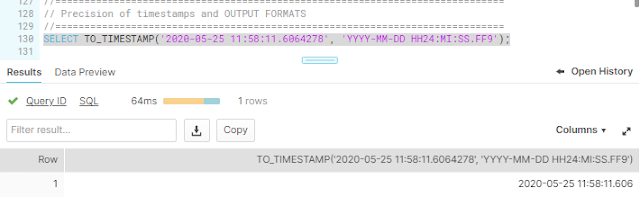`
The precision of the timestamp is still 3. Internally the Timestamp datatype is stored with precision of 9, but the milliseconds are presented with a precision of 3. You can change this with Account or sesssion parameter TIMESTAMP_NTZ_OUTPUT_FORMAT.

```ALTER SESSION SET TIMESTAMP_NTZ_OUTPUT_FORMAT = 'YYYY-MM-DD HH24:MI:SS.FF9'; SELECT TO_TIMESTAMP('2020-05-25 11:58:11.6064278', 'YYYY-MM-DD HH24:MI:SS.FF9'); Result :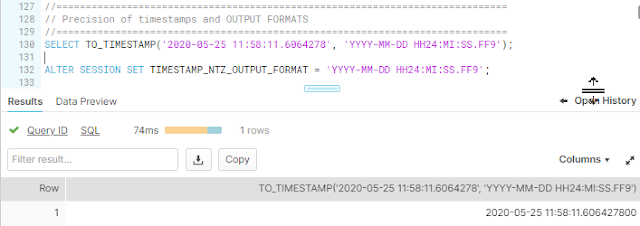The precision is now 9.And we can go back to the defaults with the UNSET command.ALTER SESSION UNSET TIMESTAMP_NTZ_OUTPUT_FORMAT; ```

## Date arithmetic

Now an example with date arithmetic.

``````SELECT CURRENT_DATE() AS D,
D + 1;```
```

Results in :

## Snowflake Date Functions

Below is a list of Snowflake Date functions with parameters, description and a possible return value.

 Date Functions Description Return SECOND (time or timestamp) Extracts the corresponding time part seconds from a time or timestamp value. 0 to 59 MINUTE (time or timestamp) Extracts the corresponding time part minute from a time or timestamp value. 0 to 59 HOUR (time or timestamp) Extracts the corresponding time part hour from a time or timestamp value. 0 to 23 DAYNAME (date or Timestamp) Extracts the three-letter day-of-week name from the specified date or timestamp Sun, Mon, Tue, Wed, Thu, Fri, Sat DAY (date or Timestamp) Extracts the corresponding date part from a date or timestamp. 1 to 31 DAYOFMONTH (date or Timestamp) Extracts the corresponding date part from a date or timestamp. 1 to 31 DAYOFYEAR (date or Timestamp) Extracts the corresponding date part from a date or timestamp. 1 to 366 WEEK (date or Timestamp) Extracts the corresponding date part from a date or timestamp. 1 to 54 WEEKISO (date or Timestamp) Extracts the corresponding date part from a date or timestamp. 1 to 53 YEAROFWEEK (date or Timestamp) Extracts the corresponding date part from a date or timestamp. Any valid year YEAROFWEEKISO (date or Timestamp) Extracts the corresponding date part from a date or timestamp. Any valid year MONTHNAME (date or Timestamp) Extracts the three-letter month name from the specified date or timestamp Jan, Feb,Mar, APR, May, Jun, Jul, Aug, Sep, Oct, Nov, Dec QUARTER (date or Timestamp) Extracts the corresponding date part from a date or timestamp. 1 to 4 YEAR (date or Timestamp) Extracts the corresponding date part from a date or timestamp. Any valid year LAST_DAY (date or time [, date_part]) Returns the last day of the specified date part for a date or timestamp. Any valid date NEXT_DAY (date_or_time_expr , dow_string) Returns the date of the first specified DOW (day of week) that occurs after the input date. Any valid date PREVIOUS_DAY (date_or_time_expr , dow_string) Returns the date of the first specified DOW (day of week) that occurs before the input date. Any valid date DATEADD ( date_or_time_part, value, date_or_time_expr) Adds the specified value for the specified date or time part to a date, time, or timestamp. DATEDIFF ( date_or_time_part, date_or_time_expr1, date_or_time_expr2) Calculates the difference between two date, time, or timestamp expressions based on the date or time part requested. Returns an integer representing the number of units (seconds, days, etc.) DATE_PART  (date_or_time_part, date_or_time_expr ) Extracts the specified date or time part from a date, time, or timestamp. EXTRACT (date_or_time_part,  FROM date_or_time_expr) Extracts the specified date or time part from a date, time, or timestamp. ADD_MONTHS ( date_or_timestamp_expr, num_months_expr) Adds or subtracts a specified number of months to a date or timestamp, preserving the end-of-month information. The data type of the returned value is the same as the data type of the first parameter. MONTHS_BETWEEN (date_expr1 , date_expr2) Returns the number of months between two DATE or TIMESTAMP values. DATE_TRUNC (date_or_time_part, date_or_time_expr) Truncates a DATE, TIME, or TIMESTAMP to the specified precision. The returned value is the same type as the input value. TIME_SLICE (date_or_time_expr, slice_length , date_or_time_part [ , start_or_end ]) Calculates the beginning or end of a “slice” of time, where the length of the slice is a multiple of a standard unit of time (minute, hour, day, etc.). DATE_FROM_PARTS( year, month, day) Creates a date from individual numeric components that represent the year, month, and day of the month. TIME_FROM_PARTS (hour, minute, second [, nanoseconds]) Creates a time from individual numeric components. TIMESTAMP_FROM_PARTS ( year, month, day, hour, minute, second [, nanosecond ] [, time_zone ] ) OR (date_expr, time_expr) Creates a timestamp from individual numeric components. If no time zone is in effect, the function can be used to create a timestamp from a date expression and a time expression.

In the nextion w'll explore the functions in more depth.

## Function SECOND()

The Snowflake function SECOND() accepts a time or a timestamp parameter and returns the seconds as an integer.

``````--Example of the Function SECOND (DATEPART in SQL SERVER/ SQL Database)
SELECT CURRENT_TIMESTAMP() AS T, SECOND(T);```
```

`Results :`

## Function MINUTE()

The Snowflake function MIINUTE() accepts a time or a timestamp parameter and returns the minutes as an integer.

``````--Example of the Function MINUTE (DATEPART in SQL SERVER/ SQL Database)
SELECT CURRENT_TIMESTAMP() AS T, MINUTE(T);```
```

`Results :`

## Function HOUR()

The Snowflake function HOUR() accepts a time or a timestamp parameter and returns the hours as an integer.

``````--Example of the Function HOUR (DATEPART in SQL SERVER/ SQL Database)
SELECT CURRENT_TIMESTAMP() AS T, HOUR(T);```
```

`Results :`

## Function DAYNAME()

Thefunction DAYNAME accepts a date or timestamp as a parameter. It will return a three letter day of week name (DOW).

``````--Example of the Function DAYNAME (DAY, DATEPART in SQL SERVER/ SQL Database)
SELECT CURRENT_TIMESTAMP() AS T, DAYNAME(T), DAYNAME(TO_DATE('2021-05-22'));```
```

`Results :`

## Function DAY ()

The function DAY() accepts date or a timestamp as a parameter and it will return an integer between 1 and 31.

``````--Example of the Function DAY (DAY,DATEPART in SQL SERVER/ SQL Database)
SELECT CURRENT_TIMESTAMP() AS T, DAY(T), DAY(TO_DATE('2021-05-22'));```
```

Results :
The DAY() Function is the same as DAYOFMONTH() and is an alternative for the usage of  the DATE_PART() (or EXTRACT) function.

## Function DAYOFMONTH()

The function DAYOFMONTH() accepts date or a timestamp as a parameter and it will return an integer between 1 and 31.

``````--Example of the Function DAYOFMONTH(T) (DATEPART in SQL SERVER/ SQL Database)
SELECT CURRENT_TIMESTAMP() AS T, DAYOFMONTH(T), DAYOFMONTH(TO_DATE('2021-05-22'));```
```

`Results :`
`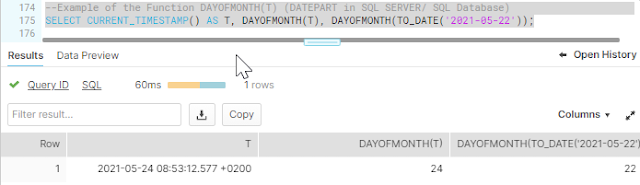`

The DAYOFMONTH() Function is the same as DAY() and is an alternative for the usage of  the DATE_PART() (or EXTRACT) function.

## Function DAYOFYEAR()

The function DAYOFYEAR() accepts date or a timestamp as a parameter and it will return an integer between 1 and 366.

``````--Example of the Function DAYOFYEAR(T) (DATEPART in SQL SERVER/ SQL Database)
SELECT CURRENT_TIMESTAMP() AS T, DAYOFYEAR(T), DAYOFYEAR(TO_DATE('2021-05-22'));```
```

`results:`
`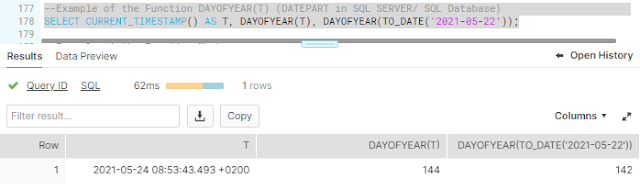`
`The DAYOFYEAR() is an alternative for the usage of  the DATE_PART() (or EXTRACT) function.`

## Function WEEK()

The function WEEK() accepts date or a timestamp as a parameter and it will return an integer between 1 and 54.

``````--Example of the Function Week (and ISO)
SELECT CURRENT_TIMESTAMP() AS T,
WEEK(T),
WEEK(TO_DATE('2021-05-22')),
WEEKISO(T),
YEAROFWEEK(T),
YEAROFWEEKISO(T);```
```

`results :`

`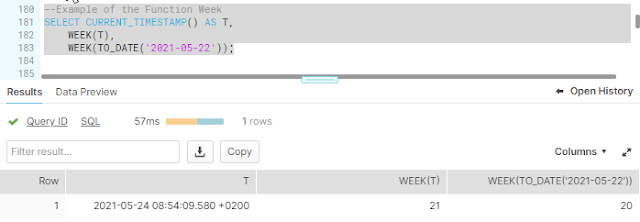`
`The WEEK() Function is the same as WEEKOFYEAR() and is an alternative for the usage of  the DATE_PART() (or EXTRACT) function.`

## Function WEEKISO()

The function WEEKISO() accepts date or a timestamp as a parameter and it will return an integer between 1 and 53.

``````SELECT CURRENT_TIMESTAMP() AS T,
WEEKISO(T);```
```

`Results : `

`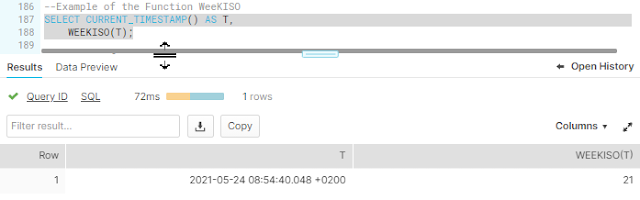The WEEKISO() Function is the same as WEEK() except it uses ISO Semantics. It is an alternative for the usage of  the DATE_PART() (or EXTRACT) function.`

## Function YEAROFWEEK()

The function YEAROFWEEK() accepts date or a timestamp as a parameter and it will return an integer, representing a valid year.

``````SELECT CURRENT_TIMESTAMP() AS T,
YEAROFWEEK(T);```
```

Results :

``

## Function YEAROFWEEKISO()

The function YEAROFWEEKISO() accepts date or a timestamp as a parameter and it will return an integer, representing a valid year.

``````SELECT CURRENT_TIMESTAMP() AS T,
YEAROFWEEKISO(T);```
```

`Results :`

`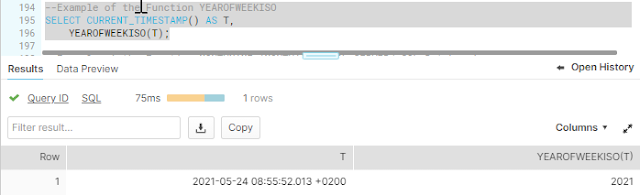`
`The YEAROFWEEKISO() Function is the same as YEAROFWEEK() except it uses ISO Semantics. It is an alternative for the usage of  the DATE_PART() (or EXTRACT) function.`

## Function MONTHNAME()

The function MONTHNAME() accepts a date or a timestamp as a parameter. It will return a three letter month name like 'May'.

```--Example of the Function MONTHNAME (MONTH() in SQL SERVER/ SQL Database)
SELECT CURRENT_TIMESTAMP() AS T, MONTHNAME(T), MONTHNAME(TO_DATE('2021-05-22'));

```

`Results :`

`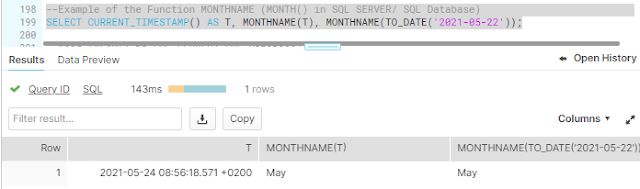`

## Function QUARTER()

The function QUARTER() accepts date or a timestamp as a parameter and it will return an integer between 1 and 4.

``````--QUARTRER (DATEPART() in SQL SERVER/ SQL Database)
SELECT CURRENT_TIMESTAMP() AS T, YEAR(T), YEAR(TO_DATE('2021-05-22'));```
```

`Results :`

## Function YEAR()

The function YEAR() accepts date or a timestamp as a parameter and it will return an integer that represents a valid year.

```--Year (YEAR() in SQL SERVER/ SQL Database)
SELECT CURRENT_TIMESTAMP() AS T, YEAR(T), YEAR(TO_DATE('2021-05-22'));
```

`Results :`
`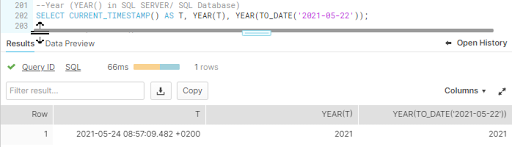`
It is an alternative for the usage of  the DATE_PART() (or EXTRACT) function.

## Function LAST_DAY()

The function LAST_DAY() accepts a date or a timestamp and an optional parameter date_part, as a parameter and it will return the last day of a date_part.

Options for the parameter date_part:
• month
• week (no weekiso)
• quarter
• year

``````--LAST_DAY (Custom logic and EOMONTH() in SQL SERVER/ SQL Database)
SELECT CURRENT_TIMESTAMP() AS T,
LAST_DAY(T),
LAST_DAY(TO_DATE('2021-05-22')),
LAST_DAY(T, 'WEEK'),
LAST_DAY(T, 'QUARTER'),
LAST_DAY(T, 'MONTH'),
LAST_DAY(T, 'YEAR');```
```

`Results :`

`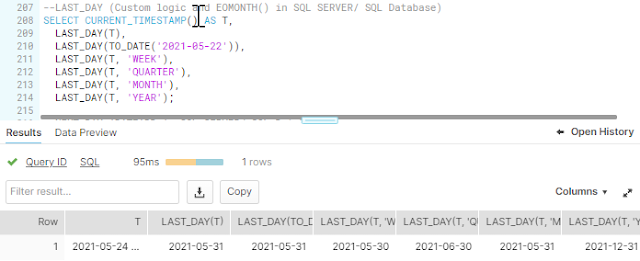`

## Function NEXT_DAY()

The function NEXT_DAY() accepts a date or a timestamp and a parameter 'day of week', as a parameter. The function will return a date value.

Valid arguments for the parameter date_part:
• sunday.
• monday.
• tuesday.
• etc.
It is also possible to enter abbreviations like 'mon'.

``````--NEXT_DAY (DATEADD in SQL SERVER/ SQL Database)
SELECT CURRENT_TIMESTAMP() AS T,
NEXT_DAY(T, 'Sunday' ),
NEXT_DAY(TO_DATE('2021-05-22'), 'Monday'),
NEXT_DAY(T, 'Tuesday'),
NEXT_DAY(T, 'we'),
NEXT_DAY(T, 'Thursday'),
NEXT_DAY(T, 'fri'),
NEXT_DAY(T, 'Saturday');```
```

`Results in :`

`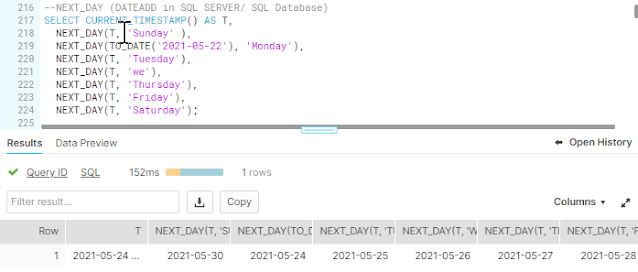`

## Function PREVIOUS_DAY ()

The function PREVIOUS_DAY() accepts a date or a timestamp and a parameter 'day of week', as a parameter. The function will return a date value.

Valid arguments for the parameter date_part:
• sunday.
• monday.
• tuesday.
• etc.
It is also possible to enter abbreviations like 'mon'.

``````--PREVIOUS_DAY (DATEADD in SQL SERVER/ SQL Database)
SELECT CURRENT_TIMESTAMP() AS T,
PREVIOUS_DAY(T, 'Sunday' ),
PREVIOUS_DAY(TO_DATE('2021-05-22'), 'Monday'),
PREVIOUS_DAY(T, 'Tuesday'),
PREVIOUS_DAY(T, 'we'),
PREVIOUS_DAY(T, 'Thursday'),
PREVIOUS_DAY(T, 'Friday'),
PREVIOUS_DAY(T, 'Saturday');```
```

`Results  :`

`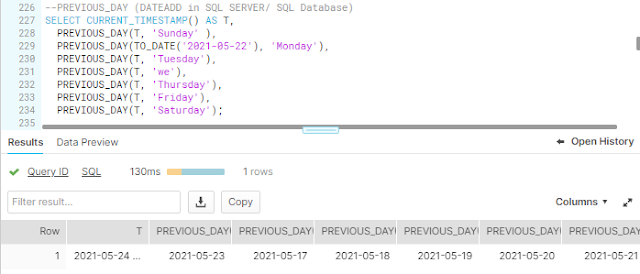`

The function DATEADD() accepts three parameters :
• a date or time part. This is the unit you would like to add. Valid arguments are year, month, day, week, quarter, hour, minute, second, millisecond, microsecond and nanosecond.
• a value. The number of units you would like to add.
• a date or a time expression.

``````--Example of the Function DATEADD (DATEADD in SQL SERVER/ SQL Database)
SELECT
CURRENT_TIMESTAMP() AS T,
```

Results (exported to a table) :

`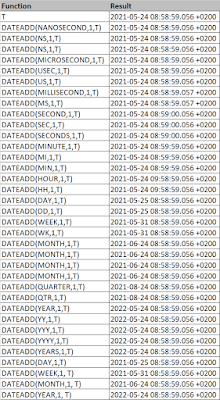`

## Function DATEDIFF ()

The function Datediff calculates the difference between two dates, time or timestamp. The function accepts three parameters :
• a date or time part. This is the unit of time in which you would like the difference.
• a date or a time expression (1).
• a date or a time expression (2).

Valid arguments for date or time part are :
• year
• month
• day
• week
• quarter
• hour
• minute
• second
• nanosecond

``````--Example of the Function DATEDIFF (DATEDIFF in SQL SERVER/ SQL Database)
--Aliasses : TIMEDIFF, TIMESTAMPDIFF
SELECT
DATEDIFF(nanosecond, column1, column2), DATEDIFF(NS,column1, column2),
DATEDIFF(ns,column1, column2),
DATEDIFF(microsecond, column1, column2), DATEDIFF(usec,column1, column2),
DATEDIFF(US,column1, column2),
DATEDIFF(millisecond,column1, column2), DATEDIFF(MS,column1, column2),
DATEDIFF(second,column1, column2), DATEDIFF(SEC,column1, column2),
DATEDIFF(SECONDS,column1, column2),
DATEDIFF(minute,column1, column2), DATEDIFF(Mi,column1, column2),
DATEDIFF(min,column1, column2),
DATEDIFF(hour,column1, column2), DATEDIFF(HH,column1, column2),
DATEDIFF(day,column1, column2), DATEDIFF(dd,column1, column2),
DATEDIFF(week,column1, column2), DATEDIFF(wk,column1, column2),
DATEDIFF(month,column1, column2), DATEDIFF(month,column1, column2),
DATEDIFF(quarter,column1, column2), DATEDIFF(qtr,column1, column2),
DATEDIFF(YEAR,column1, column2), DATEDIFF(YY,column1, column2),
DATEDIFF(YYY,column1, column2), DATEDIFF(YYYY,column1, column2),
DATEDIFF(Years,column1, column2)
FROM VALUES (TO_DATE('2020-07-17'), CURRENT_TIMESTAMP());```
```

`Results (exported to a table) :`

`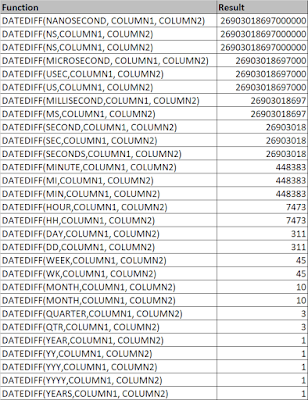`

## Function DATE_PART  ()

The function DATE_PART() (in SQL Server DATEPART()!) accepts two parameters :
• a date or time part.
• a date or a time expression.

Valid arguments for the parameter date or time part:
• year
• month
• day
• dayofweek
• dayofweekiso
• dayofyear
• week
• weekiso
• quarter
• yearofweek
• yearofweekiso
• hour
• minute
• second
• nanosecond
• epoch_second
• epoch_millisecond
• epoch_microsecond
• epoch_nanosecond
• timezone_hour
• timezone_minute

It is not possible to use the date parts millisecond and microsecond.

``````--Example of the Function DATEPART (DATEPART in SQL SERVER/ SQL Database)
SELECT
CURRENT_TIMESTAMP() AS T,
DATE_PART(epoch, T), DATE_PART(epoch_seconds,T),
DATE_PART(epoch_microsecond,T), DATE_PART(epoch_microseconds,T),
DATE_PART(epoch_nanosecond,T), DATE_PART(epoch_nanoseconds,T),
--   DATE_PART(microsecond,T), DATE_PART(usec,T), DATE_PART(US,T),
--   DATE_PART(millisecond,T), DATE_PART(MS,T),
DATE_PART(second,T), DATE_PART(SEC,T), DATE_PART(SECONDS,T),
DATE_PART(minute,T), DATE_PART(Mi,T), DATE_PART(min,T),
DATE_PART(hour,T), DATE_PART(HH,T),
DATE_PART(day,T), DATE_PART(dd,T),
DATE_PART(week,T), DATE_PART(wk,T),
DATE_PART(month,T), DATE_PART(month,T),
DATE_PART(quarter,T), DATE_PART(qtr,T),
DATE_PART(YEAR,T), DATE_PART(YY,T), DATE_PART(YYY,T), DATE_PART(YYYY,T), DATE_PART(Years,T);```
```

`Results (exported to a table) :`

`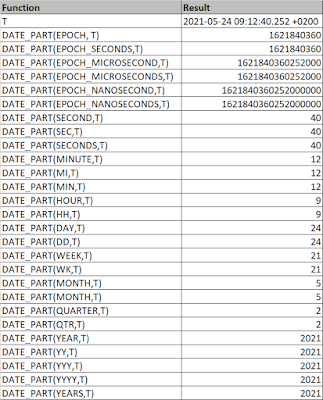`

## Function EXTRACT ()

The function EXTRACT()  accepts two parameters :
• a date or time part. This is the unit you would like to add. Valid arguments are year, month, day, week, quarter, hour, minute, second, millisecond, microsecond and nanosecond.
• a date or a time expression.

EXTRACT() is an alternative for the function DATE_PART().

``````--Example of the Function EXTRACT (DATEPART in SQL SERVER/ SQL Database)
SELECT
CURRENT_TIMESTAMP() AS T,
EXTRACT(nanosecond FROM T), EXTRACT(NS FROM T), EXTRACT(ns FROM T),
EXTRACT(epoch FROM T), DATE_PART(epoch_seconds FROM T),
EXTRACT(epoch_microsecond FROM T), EXTRACT(epoch_microseconds FROM T),
EXTRACT(epoch_nanosecond FROM T), EXTRACT(epoch_nanoseconds FROM T),
--   EXTRACT(microsecond FROM T), EXTRACT(usec FROM T), EXTRACT(US FROM T),
--   EXTRACT(millisecond FROM T), EXTRACT(MS FROM T),
EXTRACT(second FROM T), EXTRACT(SEC FROM T), EXTRACT(SECONDS FROM T),
EXTRACT(minute FROM T), EXTRACT(Mi FROM T), EXTRACT(min FROM T),
EXTRACT(hour FROM T), EXTRACT(HH FROM T),
EXTRACT(day FROM T), EXTRACT(dd FROM T),
EXTRACT(week FROM T), EXTRACT(wk FROM T),
EXTRACT(month FROM T), EXTRACT(mon FROM T),
EXTRACT(quarter FROM T), EXTRACT(qtr FROM T),
EXTRACT(YEAR FROM T), EXTRACT(YY FROM T), EXTRACT(YYY FROM T),
EXTRACT(YYYY FROM T), EXTRACT(Years FROM T);```
```

Results (exported to a table) :

`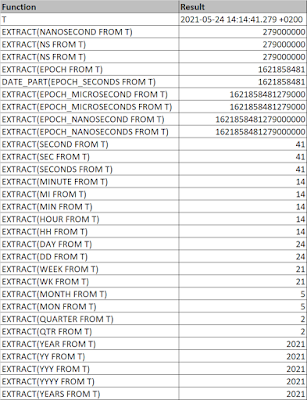`

The function ADD_MONTHS() adds or subtracts a number of months and it will preserve the end of the month information.The function accepts two parameters :
• a date or a time expression.
• number of months.

``````--Example of the Function ADDMONTHS
SELECT
CURRENT_TIMESTAMP() AS T,
```

`Results in :`

`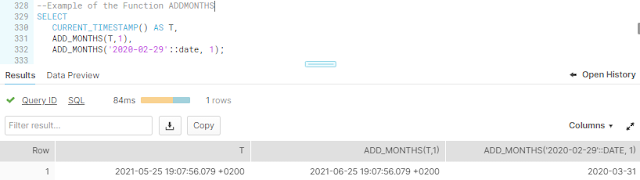`

## Function MONTHS_BETWEEN ()

The function MONTHS_BETWEEN() returns the number of months between two dates or timestamps. The function accepts two parameters :
• a date or a timestamp expression (1).
• a date or a timestamp expression (2).

An alternative is DATEDIFF()

``````--Example of the Function MONTHS_BETWEEN (DATEDIFF in SQL SERVER/ SQL Database)
SELECT
CURRENT_TIMESTAMP() AS T,
MONTHS_BETWEEN(T,TO_DATE('2020-07-17')),
DATEDIFF(month,T,TO_DATE('2020-07-17'));```
```

`Results in :`

`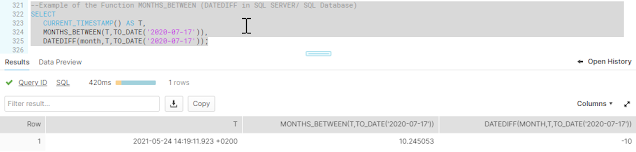`

## Function DATE_TRUNC ()

The function DATE_TRUNC() truncates a date or time expression to a certain specified precision. The function accepts two parameters :
• a date or a time part.
• a date or a timestamp expression.

Valid arguments for date or time part are :
• year
• month
• day
• week
• quarter
• hour
• minute
• second
• nanosecond

``````-- Example of the Function DATE_TRUNC (CAST/CONVERT and custom logic in SQL SERVER/ SQL Database)
-- Aliasses TRUNC
SELECT
CURRENT_TIMESTAMP() AS T,
DATE_TRUNC(nanosecond, T), DATE_TRUNC(NS, T), DATE_TRUNC(ns, T),
DATE_TRUNC(microsecond, T), DATE_TRUNC(usec, T), DATE_TRUNC(US, T),
DATE_TRUNC(millisecond, T), DATE_TRUNC(MS, T),
DATE_TRUNC(second, T), DATE_TRUNC(SEC, T), DATE_TRUNC(SECONDS, T),
DATE_TRUNC(minute,T), DATE_TRUNC(MI,T), DATE_TRUNC(min,T),
DATE_TRUNC(hour, T), DATE_TRUNC(HH, T),
DATE_TRUNC(day, T), DATE_TRUNC(dd,T),
DATE_TRUNC(week, T), DATE_TRUNC(wk, T),
DATE_TRUNC(month, T), DATE_TRUNC(month, T),
DATE_TRUNC(quarter, T), DATE_TRUNC(qtr, T),
DATE_TRUNC(YEAR, T), DATE_TRUNC(YY, T), DATE_TRUNC(YYY, T),
DATE_TRUNC(YYYY, T), DATE_TRUNC(Years, T),
DATE_TRUNC(HOUR,T),
DATE_TRUNC(month,T);  ```
```

Results (exported to a table) :

`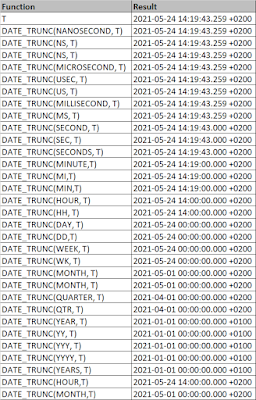`

## Function TIME_SLICE ()

The function TIME_SLICE calculates the beginning or the end of a slice.  This function can be used to calculate start and ends of fixed buckets into which datea can be categorized. This function accepts four parameters:
• a date or time expression
• The slice length
• the date or time part
• START or END

``````-- Example of the Function TIME_SLICE (? in SQL SERVER/ SQL Database)
SELECT
CURRENT_TIMESTAMP()::timestamp_ntz  AS T,
TIME_SLICE(T, 4, 'MINUTE', 'START'),
TIME_SLICE(T, 4, 'MINUTE', 'END'),
TIME_SLICE(T, 4, 'HOUR', 'START'),
TIME_SLICE(T, 4, 'HOUR', 'END'),
TIME_SLICE(T, 4, 'DAY', 'START'),
TIME_SLICE(T, 4, 'DAY', 'END'),
TIME_SLICE(T, 4, 'WEEK', 'START'),
TIME_SLICE(T, 4, 'WEEK', 'END'),
TIME_SLICE(T, 4, 'QUARTER', 'START'),
TIME_SLICE(T, 4, 'QUARTER', 'END'),
TIME_SLICE(T, 4, 'YEAR', 'START'),
TIME_SLICE(T, 4, 'YEAR', 'END');```
```

`Results (exported to a table) :`

`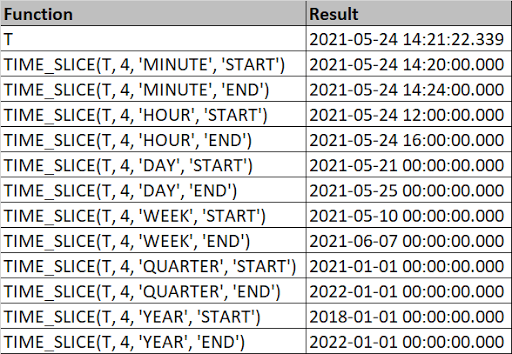`

## Function DATE_FROM_PARTS()

The function DATE_FROM_PARTS() creates a date from individual numeric components that represents a year, month and the day of the month. The function accepts three parameters :
• year
• month
• day

``````-- Example of the Date_From_Parts Functions (DATEFROMPARTS in SQL SERVER/ SQL Database)
SELECT
DATE_FROM_PARTS(2021,5,22),
DATE_FROM_PARTS(2021, 5, 100),
DATE_FROM_PARTS(2021, 1 + 24, 1),
DATE_FROM_PARTS(2021, -1, -1);```
```

`Results in :`

`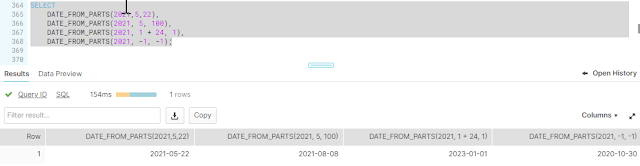`

## Function TIME_FROM_PARTS ()

The function TIME_FROM_PARTS() creates a date from individual numeric components that represents a hour, minute, second and nanoseconds (optional) . The function accepts four parameters :
• hour
• minutes
• seconds
• nanoseconds

``````-- Example of the Time_From_Parts Functions (DATETIMEFROMPARTS, CAST in SQL SERVER/ SQL Database)
SELECT
TIME_FROM_PARTS(20, 20, 22,12323),
TIME_FROM_PARTS(20,1000,22,12323);```
```

`Results in :`

`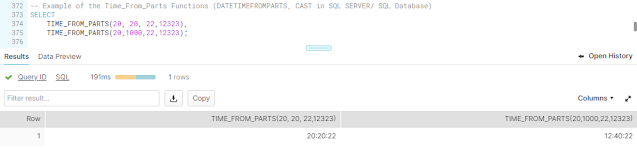`

## Function TIMESTAMP_FROM_PARTS ()

The function TIMESTAMP_FROM_PARTS() creates a timestamp from individual numeric components  or a date and time expression. The first variant has  8 parameters and the second variant two parameters. The first variant uses the following parameters :
• year
• month
• day
• hour
• minute
• second
• nanosecond (optional)
• timezone
The second variant accept two parameters :
• date expression
• time expression

``````-- Example of the Timestamp_From_Parts Functions (DATETIMEFROMPARTS  in SQL SERVER/ SQL Database)
SELECT
TIMESTAMP_FROM_PARTS(2021,5,22, 20,20,22,12323),
TIMESTAMP_FROM_PARTS(2021,5,22,20,1000,22,12323),
TIMESTAMP_FROM_PARTS('2021-05-23', '12:00:00'),
TIMESTAMP_LTZ_FROM_PARTS(2021,5,22, 20,20,22,12323),
TIMESTAMP_LTZ_FROM_PARTS(2021,5,22,20,1000,22,12323),
TIMESTAMP_NTZ_FROM_PARTS(2021,5,22, 20,20,22,12323),
TIMESTAMP_NTZ_FROM_PARTS(2021,5,22,20,1000,22,12323),
TIMESTAMP_TZ_FROM_PARTS(2021,5,22, 20,20,22,12323),
TIMESTAMP_TZ_FROM_PARTS(2021,5,22,20,1000,22,12323),
TIMESTAMP_TZ_FROM_PARTS(2021,5,22,20,1000,22,12323, 'America/Los_Angeles');```
```

`Results (exported to a table) :`

`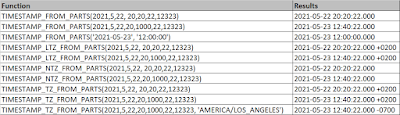`

## Final Thoughts

This was a blogpost about Snowflake functions and I hope you enjoyed it.

Hennie